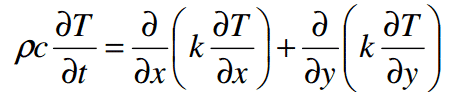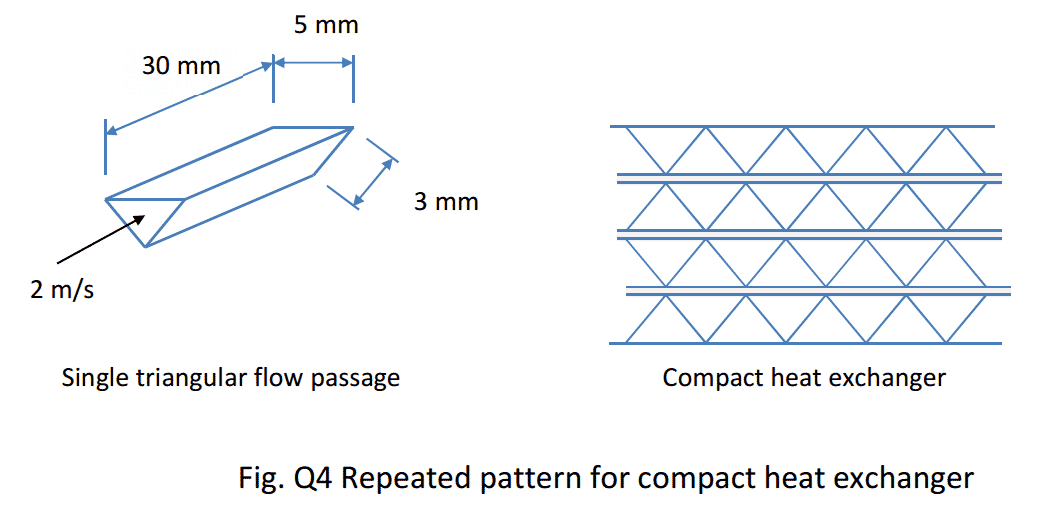# Thermodynamics Assignment Help

Are you in need of thermodynamics/ heat and mass transfer assignment help? Order your thermodynamics assignment help now. The following are sample thermodynamics questions:

Q. 1 (1 mark) A cooling fin for a microchip is subjected to a 1.5 m/s air velocity parallel to the surface. The fin is 1.5 cm high and 3 cm wide with both sides of the fin exposed to the same air velocity. If the surface temperature is 65 °C and the air temperature is 17 °C, determine the rate of heat loss via forced convection from the fin.Q. 2 (1 mark) The unsteady 2D heat conduction equation is given by:where T is the temperature, ρ is the density, c is the specific heat capacity, and k is the thermal conductivity. Derive this equation from first principles by applying energy conservation to a control volume in the shape of a rectangle, making use of Fourier’s law of conduction and
a Taylor series expansion

Q. 3 (2 marks) For a semi-infinite solid with a constant surface temperature Ts and initial temperature Ti, the temperature at any point beneath the surface is given by:as a function of time, where erf() is the error function, x is the distance from the surface, t is time and α is the thermal diffusivity. Show that:
a) Eq. (2) satisfies the 3D unsteady heat conduction equation, Eq. (3) and b) Eq. (2) also satisfies the boundary conditions given by Equations (4) and (5).Q. 4 (2 marks)

Compact heat exchanger s often make use of a repeating pattern of fins to create a large surface area to enhance heat transfer. Figure Q4 shows a pattern of triangular flow passages for the air side of the heat exchanger. Assuming the temperature of the fins is constant over the entire fin, determine the average heat transfer coefficient for air flowing at 2m/s through a single flow passage.Q. 5 (2 marks) A cross-flow heat exchanger with both streams unmixed is used as a preheater to heat up air (initially at 25 °C) for a combustion process using hot exhaust gas at 200°C (assume properties of air). The flow rate of air on the cold side of the exchanger is 2 kg/s.
On the hot side of the exchanger the mass flow rate is 2.1 kg/s. The heat transfer surface in the heat exchanger has an area of 25 m2 and the overall heat transfer coefficient is 140W/m2.K. Determine the temperature of the air on the cold side as it exits the pre-heater.

Q. 6 (2 marks) A 70 W tungsten lightbulb filament has a length of 60 cm and a diameter of 50µm. The transparent glass bulb housing the filament holds a sufficiently high vacuum to justify neglecting free convection. Assume the electrical resistivity of the tungsten is 6 × 10-8 Ω⋅m, the emissivity of the wire surface is 0.25 (for all wavelengths) and the temperature of the room is
25 °C.
Determine:
a) The temperature of the tungsten filament when the electrical current is 2 amps.
b) The power in Watts of radiation emitted in the visible spectrum.